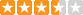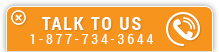# Statistical Process Simulation - A Linear Regression Approach

Course Number: I-3003
Credit: 3 PDH
Subject Matter Expert: Manuel Calzada, P.E.
Price: \$89.85 Purchase using Reward Tokens. Details8 reviews
Overview

#### In Statistical Process Simulation - A Linear Regression Approach, you'll learn ...

• The purpose of process simulation
• The difference between the theoretical method and the statistical method and when to use one method or the other
• The methodology for designing a real experiment using the statistical method
• What is the Significant F number and why is it important
• How to use a Relative Weights procedure for optimization

#### OverviewPreview a portion of this course before purchasing it.

Credit: 3 PDH

Length: 30 pages

Simulation processes are techniques which are used to estimate, predict and forecast behaviors. These tools and techniques can be extremely helpful and can be used as tools to design, reduce cost and time, improve success and / or reduce risks.

This course is designed to illustrate the use of different techniques that can be used to simulate processes. Some processes are well known and there are many accurate mathematical equations that can help to predict the performance of a process with a fairly high degree of accuracy. We will call these Theoretical Methods.

Unlike the theoretical methods, other processes are not as clear or don’t have mathematical equations that can help predict the behavior of some variables in the process. In these cases we have to use experience or experimentation to develop such equations so we can simulate their behavior. This second method will be called Statistical Method.

This course will concentrate only on the Statistical Method.

#### Specific Knowledge or Skill Obtained

This course teaches the following specific knowledge and skills:

• Understand the purpose of process simulation
• Understand different ways of simulating processes
• Basics of a statistical process simulation and procedure to solve complex problems
• Linear regression techniques for single and multiple variable cases
• Become familiar with Microsoft Excel statistical tools
• Develop linear regression formulas and analyze results
• The relationship between correlation of variables and causation
• What is an interaction of resources and how it affects the outputs
• Optimize results using Microsoft Excel tools

#### Certificate of Completion

You will be able to immediately print a certificate of completion after passing a multiple-choice quiz consisting of 15 questions. PDH credits are not awarded until the course is completed and quiz is passed.

Board Acceptance
 This course is applicable to professional engineers in: Alabama (P.E.) Alaska (P.E.) Arkansas (P.E.) Delaware (P.E.) Florida (P.E. Area of Practice) Georgia (P.E.) Idaho (P.E.) Illinois (P.E.) Illinois (S.E.) Indiana (P.E.) Iowa (P.E.) Kansas (P.E.) Kentucky (P.E.) Louisiana (P.E.) Maine (P.E.) Maryland (P.E.) Michigan (P.E.) Minnesota (P.E.) Mississippi (P.E.) Missouri (P.E.) Montana (P.E.) Nebraska (P.E.) Nevada (P.E.) New Hampshire (P.E.) New Jersey (P.E.) New Mexico (P.E.) New York (P.E.) North Carolina (P.E.) North Dakota (P.E.) Ohio (P.E. Self-Paced) Oklahoma (P.E.) Oregon (P.E.) Pennsylvania (P.E.) South Carolina (P.E.) South Dakota (P.E.) Tennessee (P.E.) Texas (P.E.) Utah (P.E.) Vermont (P.E.) Virginia (P.E.) West Virginia (P.E.) Wisconsin (P.E.) Wyoming (P.E.)
Reviews (8)
More DetailsPreview a portion of this course before purchasing it.

Credit: 3 PDH

Length: 30 pages